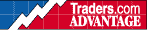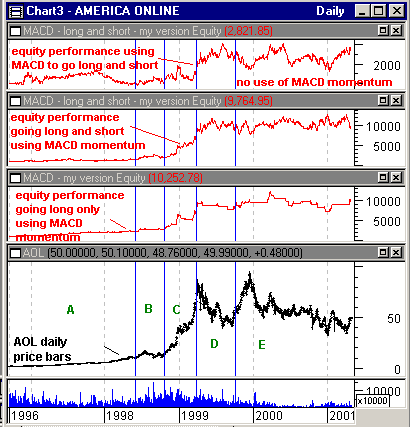HOT TOPICS LIST

INDICATORS LIST

LIST OF TOPICS

# MACD (Part II Of II)

05/11/01 02:36:31 PM
by Dennis D. Peterson

Analyzing how MACD works makes a good case study for many indicators.

Security:   AOL
Position:   N/A

 What are the characteristics of a trend? First, prices fall faster than they rise. Second, the most common continuation pattern seen in a trend is a wedge. You are concerned with trend behavior because MACD uses moving averages which are trend-following devices. If the periods you have chosen for MACD work well for an uptrend, would they work as well for a downtrend? Given that the price dynamics are different between rising and falling prices, momentum should behave differently as well. If you go back to the histogram in Figure 1 of Part I, you will see that the greatest changes of momentum are on the negative side. This leads to the notion that you should trade with MACD only when it is above zero if the default periods are working well for uptrends.From Part I, recall that using a 50-day moving average to generate long and short positions resulted in \$1,000 invested in '96 growing to \$5,000.Figure 1: Equity performance for three different trading systems trading daily AOL prices. Top chart uses MACD alone for long and short positions. Second chart from top uses MACD and MACD momentum for long and short positions. Third chart from top uses MACD and MACD momentum and only takes long positions. Bottom chart is AOL daily price and volume data. Graphic provided by: MetaStock. Graphic provided by: Data vendor: eSignal<. Figure 1 shows the equity performance of three different trading systems. The top chart is the result of \$1,000 invested in '96 using the following trading system: go long if PO (see Part I -- PO and the moving average of PO are the same as MACD and its signal line) is above its moving average, and go short if PO is below its signal line. I have divided Figure 1 into five sections. You can see that section C had the best price gain.MACD has problems when it hits a wedge. Going back to Figure 1 of Part I, you will see that areas B and C, where MACD was flat, are areas where wedges occurred while in a trend. From a trend-following indicator standpoint wedges are unwelcome sights. From a technician's viewpoint they are welcomed sights because there is a degree of predictability to them - a certain volume behavior. MACD doesn't use volume and is therefore running at a handicap. If you read "Technical Analysis of Stock Trends" by Robert Edwards and John Magee you will find that volume plays a major role.However, MACD does have some redeeming qualities. If I use MACD momentum in conjunction with MACD, I can make some adjustments for wedges. When a continuation wedge pattern is encountered, the momentum is going to decrease because in an uptrend the falling wedge is the continuation pattern, while in a downtrend the rising wedge is the continuation pattern. In other words I should be able to minimize the effect of wedges by using MACD momentum, that is the histogram.Here's the other question: if I substantially improve MACD performance using MACD momentum for long and short positions, and I have optimized the moving average periods that do well for long and short positions, would I see a difference if I only took long positions? In other words, would I see that using momentum of MACD for long and short entry only made losses smaller at the expense of smaller gains and a lot more trades being committed -- still whipsawed into trading commission purgatory? The top chart used a trading system that goes long if PO is greater than the moving average of PO, and goes short if PO is less than its moving average. In other words, if MACD is above its signal line, go long and if it's below go short. The result is that it didn't beat a simple moving average. I'll now add MACD momentum. The second chart from the top added the condition that the moving average of the difference of PO and moving average of PO be positive to go long. This is the equivalent of asking: Is the histogram above zero? The Metastock equation is:Mov(diff-signal,3,E)>0, where "Mov" means moving average, "diff-signal" is the difference of PO and its moving average ("diff " is the difference of the two moving averages and "signal" is moving average of the difference of the two moving averages), the "3" means three periods and "E" means exponential moving average. The full set of Metastock equations for long are:diff:=Mov(C,opt1,E)-Mov(C,opt2,E); signal:=Mov(diff,opt3,E);cond1:=diff>signal;cond2:= diff>0;cond3:=Mov(diff-signal,3,E)>0;cond1 AND cond2 AND cond3 The values that the system reached for opt1, opt2, and opt3 are 16, 34, and 4, respectively. The last statement "cond1 AND cond2 AND cond3" means go long if "cond1" is true, "cond2" is true, and "cond3" is true. For example "cond1" is true if the difference of the moving averages, "diff", is greater than the moving average of the differences, "signal." So, is MACD above its signal line? The equations for going short are the same, EXCEPT I removed cond2 and made cond1 read diff < signal. I wanted PO below its signal line, except it isn't necessary to have PO negative. The other observation was that downtrends generally started when MACD momentum was positive. In other words, I wanted three conditions to be true before I went long: positive MACD momentum, MACD above its signal line, and MACD above zero. I made going short very easy by having the MACD go below its signal line EVEN when MACD momentum is positive. So my solution for not having periods that would automatically adjust by being in an uptrend or downtrend was to be patient getting in long, and then go short as soon as I could.You can see a dramatic improvement in equity performance by including MACD momentum. An investment of \$1,000 in '96 now results in \$10,000 today and the moving average system has been beaten.But wouldn't a better system be to have the MACD momentum be negative to take a short position? The answer is that trading system ends up with \$1,000 not counting the commissions! What about implementing a trading system that goes short when MACD momentum goes negative and MACD is below its signal line - the mirror image of the conditions for going long? That system ends up at \$20,000! But wait, that system makes five trades in the entire five years. The equity curve matches the price curve. It's the same as buy-and-hold. You don't need any kind of trading system if you pick a stock worth \$2 per share years ago and it's now worth \$50 per share. What you want is a system that builds equity and hopefully hangs on to it, and isn't necessarily the long term success story of AOL.The third equity chart from the top only takes long positions. I also optimized, and using the same formulas above the values for the two moving averages and the signal line are opt1=8, opt2=20, and opt3=3, respectively. I used the rule to exit as fast as I could so when PO is less than the moving average of PO, I exit. This occurs as soon as MACD is below its signal line, EVEN if the momentum of MACD is positive (histogram above zero). So when I optimize for going long only, I get a different set of periods for MACD than when I optimize for going both long and short. This only confirms that periods optimized for uptrends reflect the slower rate of change. Downtrends accelerate faster than uptrends, as seen in the histogram. When you look at the price history of AOL and areas A, B, C, D, and E, you'll see the full gambit of price action. Trend-following systems loathe chop. The last three-fourths of area E is chop, and MACD didn't do well. Area B is mild chop. Area A is a gradual uptrend. Area D is a downtrend - so why didn't MACD shorts work better there? From an optimization standpoint AOL went from \$2/share to \$50/share and any optimized values are going to be biased for this overall gain. You can see the effect rather dramatically with the equity growth using MACD and momentum for long and shorts for the peak at the beginning of area E. Equity grew on the uptrend but decreased with the downtrend. Now you say, if a stock is in a downtrend, then optimize for the downtrend. But, if you are going to do that then why do you need MACD?The number of trades to go long only (Figure 1: third chart from top) were 45; for going both long and short there were 94 (Figure 1: second chart from top). If you are paying \$10 per trade, no big deal. But half of the 94 long and short trading system trades were in area A, just when you are trying to build some equity. Area A is an accumulation period. If you ever see it, get in and do some accumulating of your own without regard to any indicator (except NVI: negative volume index). I would suggest the following rules for using MACD:1. Use the default periods of 12, 26, and 9 for the periods of the two moving averages of PO and the moving average of PO respectively, that is, MACD and its signal line.2. Use MACD for long positions only.3. Take a long position when PO is positive (MACD above the zero line), the histogram is above zero and PO is greater than its moving average (MACD above its signal line).4. Exit as soon as PO goes below its average (MACD is below its signal line), regardless of anything else. You will probably get whipsawed a bit with this rule, but you are better off preserving your capital with losses only due to commissions.

Dennis D. Peterson

Market index trading on a daily basis.

 Comments or Questions? Article Usefulness 5 (most useful) 4 3 2 1 (least useful)

Date:�05/15/01Rank:�5Comment:
Date:�12/29/01Rank:�5Comment:
Date:�07/01/03Rank:�4Comment: